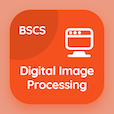Business Statistics Quiz PDF - Complete

# Data Classification Quiz MCQ Online p. 15

Practice Data Classification quiz questions and answers PDF, data classification trivia questions 15 to learn online Business Statistics course for online classes. Data Classification, Tabulation and Presentation MCQ questions, data classification Multiple Choice Questions (MCQ) for online college degrees. "Data Classification Quiz" PDF eBook: classification: measures of dispersion, probability experiments, average deviation measures, binomial distribution, data classification test prep for online business university.

"The largest value is 60 and the smallest value is 40 and the number of classes desired is 5 then the class interval is" MCQ PDF: 4, 20, 25, and 15 for online business and administration degree. Solve data classification, tabulation and presentation questions and answers to improve problem solving skills for online business administration degree.

## Trivia Quiz on Data Classification MCQs

MCQ: The largest value is 60 and the smallest value is 40 and the number of classes desired is 5 then the class interval is

20
4
25
15

MCQ: If the value of p is smaller or lesser than 0.5 then the binomial distribution is classified as

skewed to right
skewed to left
skewed to infinity
skewed to integers

MCQ: The number of patients who visited the cardiologists are as 63, 57, 51, 65 in four days then the absolute mean deviation (approximately) is

8 patents
4 patients
10 patients
15 patients

MCQ: The number of individuals arriving at boarding counter on an airport is an example of

numerical outcome
non numerical outcome
random outcome
simple outcome

MCQ: In terms of dispersion difference, the measurement of dispersion for available data is classified as

average measures
distance measures
average deviation measures
availability measures

### More Quizzes from Business Statistics Course